#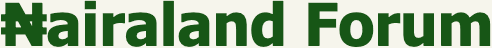Welcome, Guest: Join Nairaland / LOGIN! / Trending / Recent / New
Stats: 2,758,710 members, 6,557,699 topics. Date: Tuesday, 26 October 2021 at 08:31 PM

## I.Q TEST: Let's See How Sound You Are In Quantitative Reasoning - Family (4) - Nairaland

(1) (2) (3) (4) (5) (Reply) (Go Down)

 Re: I.Q TEST: Let's See How Sound You Are In Quantitative Reasoning by veave(f): 9:44pm On Apr 12, 2020 matrix199:I'll reveal the answers to pix 1 and pix 2 and acknowledge those who got it right later on. cc: RoyalRoyModified: I'm a very discreet person. The term 'acknowledge' means more than you might think. You never can tell! Puzzle 1For picture 1; let x represents the helmet. It implies that x + x + x = 30 3x = 30 x = 30/3 = 10 so x is 10For picture 2, let y represents the car. It implies that y + y+ x = 20 since x is 10 y + y + 10 = 20 2y + 10 = 20 2y = 20 - 10 2y = 10 y = 10/2 = 5 so y is 5For picture 3, let z represents the pair of trophies( 2 trophies). It implies that z + z + y = 13 since y is 5 z + z + 5 = 13 2z + 5 = 13 2z = 13 - 5 2z = 8 z = 8/2 = 4 so z is 4 But remember that z is a pair of trophies It implies that 1 trophy is 4/2 = 2For Picture 4, x(10) + y(5) x 1/2 of z(2) _____ 10 + 5 x 2Apply BODMAS _______________ 10 + (5 x 2) 10 + 10 = 20. Puzzle 2Take a good look at each quantitative picture. The 1st picture clearly shows 7 petals in each flower pot(7 + 7 + 7 = 21), discreetly indicating that each petal is 1. The 2nd picture also shows 4 roses in each flower pot. it implies that ( 4x2 + 4x2 + 4x2 =8 + 8 + 8= 24 or 12x2 = 24). The 3rd picture indicates that each empty pot is 4, that is ( 4 + 4 + 4 = 12). Now, the 4th picture shows a flower pot of 6 petals( each petal is 1, totalling 6 in combined value) + an empty flower pot having a value of 4 x a flower pot of 5 roses having a combined value of 10(since each rose is 2 in value) That is, 6 + 4 x 10 Don't forget to apply "BODMAS" 6 + (4 x 10) 6 + 40 = 46. Thus, for puzzle 1, the answer is 20! for puzzle 2, the answer is 46!This is incorrect. 1 Like Re: I.Q TEST: Let's See How Sound You Are In Quantitative Reasoning by Epositive(m): 9:46pm On Apr 12, 2020 matrix199:Take it easy young man or lady. Guess what, in the eyes of a monkey, humans are monkeys.In regards to the second puzzle. How about the empty flower pot, I thought it's doubled? I think you should mention the owner of this puzzle. We will not take this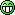1 Like Re: I.Q TEST: Let's See How Sound You Are In Quantitative Reasoning by matrix199(m): 9:47pm On Apr 12, 2020 veave:This is incorrect. Check picture 1. What can you see?Check picture 3. What can you see? Re: I.Q TEST: Let's See How Sound You Are In Quantitative Reasoning by veave(f): 9:47pm On Apr 12, 2020 matrix199:Check picture 1. What can you see?Check picture 3. What can you see?Picture one is 40 not 20 1 Like Re: I.Q TEST: Let's See How Sound You Are In Quantitative Reasoning by matrix199(m): 9:53pm On Apr 12, 2020 Epositive:In regards to the second puzzle. How about the empty flower pot, I thought it's doubled? I think you should mention the owner of this puzzle. We will not take thisIt's a play of trick. That the pot has two ring rigs doesn't allude it's doubled. The point is about the value of its emptiness. The alteration in the 4th picture of the empty pot was to create skepticism Re: I.Q TEST: Let's See How Sound You Are In Quantitative Reasoning by Mechatronika(m): 9:53pm On Apr 12, 2020 matrix199: Can I lead you through it, or would you lead? You're the maaaan! you do it.Re: I.Q TEST: Let's See How Sound You Are In Quantitative Reasoning by Mechatronika(m): 9:56pm On Apr 12, 2020 matrix199:It's a play of trick. That the pot has two ring rigs doesn't allude it's doubled. The point is about the value of its emptiness. The alteration in the 4th picture of the empty pot was to create skepticism Don't gimme this shit! 1 Like Re: I.Q TEST: Let's See How Sound You Are In Quantitative Reasoning by Epositive(m): 9:59pm On Apr 12, 2020 matrix199:It's a play of trick. That the pot has two ring rigs doesn't allude it's doubled. The point is about the value of its emptiness. The alteration in the 4th picture of the empty pot was to create skepticism And the pot of petals too. You ignored the pot and calculated only the petals. We wouldn't have possibly ignored the pot when there are values for empty pots and petals in picture 3 and 1 respectively. Any explanation for that? Re: I.Q TEST: Let's See How Sound You Are In Quantitative Reasoning by everydayboy: 10:01pm On Apr 12, 2020 1) 402) 90Best regards.... Re: I.Q TEST: Let's See How Sound You Are In Quantitative Reasoning by Mechatronika(m): 10:02pm On Apr 12, 2020 Here is a Hint; The question; (Rose+Pot) + (2pots) x (flower + pot) Now, find the values for the parameters and solve. Re: I.Q TEST: Let's See How Sound You Are In Quantitative Reasoning by matrix199(m): 10:04pm On Apr 12, 2020 Mechatronika:Don't gimme this shit!Men In Black 1, Who would have thought that a little girl would be used as a test. But only one who reasoned outside the box figured out the unusual presence of a child in an unusual place.That's the essence of such test. Why was this car helmetted? Why was this pot double ring rigged? To make that split seconds choice if it's Significant or insignificant Re: I.Q TEST: Let's See How Sound You Are In Quantitative Reasoning by matrix199(m): 10:08pm On Apr 12, 2020 Epositive:And the pot of petals too. You ignored the pot and calculated only the petals. We wouldn't have possibly ignored the pot when there are values for the empty pot and petals in picture 1 and 3 respectively. Any explanation for that? Jesus is in for it when he returns. These ones here are going to question him to death when he returns. Re: I.Q TEST: Let's See How Sound You Are In Quantitative Reasoning by Spechialone(f): 10:08pm On Apr 12, 2020 Epositive:I'm disappointed. To even think that I've been waiting for the solution all this while.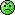upon all our waiting ... Re: I.Q TEST: Let's See How Sound You Are In Quantitative Reasoning by Spechialone(f): 10:10pm On Apr 12, 2020 veave: Picture one is 40 not 20 yes, tell him. Lol Re: I.Q TEST: Let's See How Sound You Are In Quantitative Reasoning by everydayboy: 10:12pm On Apr 12, 2020 matrix199:I'll reveal the answers to pix 1 and pix 2 and acknowledge those who got it right later on. cc: RoyalRoyModified: I'm a very discreet person. The term 'acknowledge' means more than you might think. You never can tell! Puzzle 1For picture 1; let x represents the helmet. It implies that x + x + x = 30 3x = 30 x = 30/3 = 10 so x is 10For picture 2, let y represents the car. It implies that y + y+ x = 20 since x is 10 y + y + 10 = 20 2y + 10 = 20 2y = 20 - 10 2y = 10 y = 10/2 = 5 so y is 5For picture 3, let z represents the pair of trophies( 2 trophies). It implies that z + z + y = 13 since y is 5 z + z + 5 = 13 2z + 5 = 13 2z = 13 - 5 2z = 8 z = 8/2 = 4 so z is 4 But remember that z is a pair of trophies It implies that 1 trophy is 4/2 = 2For Picture 4, x(10) + y(5) x 1/2 of z(2) _____ 10 + 5 x 2Apply BODMAS _______________ 10 + (5 x 2) 10 + 10 = 20. Puzzle 2Take a good look at each quantitative picture. The 1st picture clearly shows 7 petals in each flower pot(7 + 7 + 7 = 21), discreetly indicating that each petal is 1. The 2nd picture also shows 4 roses in each flower pot. it implies that ( 4x2 + 4x2 + 4x2 =8 + 8 + 8= 24 or 12x2 = 24). The 3rd picture indicates that each empty pot is 4, that is ( 4 + 4 + 4 = 12). Now, the 4th picture shows a flower pot of 6 petals( each petal is 1, totalling 6 in combined value) + an empty flower pot having a value of 4 x a flower pot of 5 roses having a combined value of 10(since each rose is 2 in value) That is, 6 + 4 x 10 Don't forget to apply "BODMAS" 6 + (4 x 10) 6 + 40 = 46. Thus, for puzzle 1, the answer is 20! for puzzle 2, the answer is 46!Lmao..... Beautiful nonsense.Lol.... No vex brother...... Re: I.Q TEST: Let's See How Sound You Are In Quantitative Reasoning by matrix199(m): 10:13pm On Apr 12, 2020 I've passed my bedtime. Let's constructively debate this tomorrow or some other time. You're all acknowledged. Thank you for your time. At least I, we survived another day of stay at home.Bon nuit! Re: I.Q TEST: Let's See How Sound You Are In Quantitative Reasoning by Spechialone(f): 10:23pm On Apr 12, 2020 matrix199:I've passed my bedtime. Let's constructively debate this tomorrow or some other time. You're all acknowledged. Thank you for your time. At least I, we survived another day of stay at home.Bon nuit! Just tell us you wanted to recruit for The SSS Re: I.Q TEST: Let's See How Sound You Are In Quantitative Reasoning by Epositive(m): 10:34pm On Apr 12, 2020 matrix199:Jesus is in for it when he returns. These ones here are going to question him to death when he returns. Answer the damn question bruhRe: I.Q TEST: Let's See How Sound You Are In Quantitative Reasoning by LadySarah: 1:37am On Apr 13, 2020 3039 Re: I.Q TEST: Let's See How Sound You Are In Quantitative Reasoning by izzygoing(f): 2:35am On Apr 13, 2020 The first puzzle = 30 The second puzzle =120 ] Re: I.Q TEST: Let's See How Sound You Are In Quantitative Reasoning by Hallenjay: 6:28am On Apr 13, 2020 matrix199:I'll reveal the answers to pix 1 and pix 2 and acknowledge those who got it right later on. cc: RoyalRoyModified: I'm a very discreet person. The term 'acknowledge' means more than you might think. You never can tell! Puzzle 1For picture 1; let x represents the helmet. It implies that x + x + x = 30 3x = 30 x = 30/3 = 10 so x is 10For picture 2, let y represents the car. It implies that y + y+ x = 20 since x is 10 y + y + 10 = 20 2y + 10 = 20 2y = 20 - 10 2y = 10 y = 10/2 = 5 so y is 5For picture 3, let z represents the pair of trophies( 2 trophies). It implies that z + z + y = 13 since y is 5 z + z + 5 = 13 2z + 5 = 13 2z = 13 - 5 2z = 8 z = 8/2 = 4 so z is 4 But remember that z is a pair of trophies It implies that 1 trophy is 4/2 = 2For Picture 4, x(10) + y(5) x 1/2 of z(2) _____ 10 + 5 x 2Apply BODMAS _______________ 10 + (5 x 2) 10 + 10 = 20. Puzzle 2Take a good look at each quantitative picture. The 1st picture clearly shows 7 petals in each flower pot(7 + 7 + 7 = 21), discreetly indicating that each petal is 1. The 2nd picture also shows 4 roses in each flower pot. it implies that ( 4x2 + 4x2 + 4x2 =8 + 8 + 8= 24 or 12x2 = 24). The 3rd picture indicates that each empty pot is 4, that is ( 4 + 4 + 4 = 12). Now, the 4th picture shows a flower pot of 6 petals( each petal is 1, totalling 6 in combined value) + an empty flower pot having a value of 4 x a flower pot of 5 roses having a combined value of 10(since each rose is 2 in value) That is, 6 + 4 x 10 Don't forget to apply "BODMAS" 6 + (4 x 10) 6 + 40 = 46. Thus, for puzzle 1, the answer is 20! for puzzle 2, the answer is 46!I said it before that the puzzle is not urs. When am less busy I'll proof it to u just remind me incase I forge Re: I.Q TEST: Let's See How Sound You Are In Quantitative Reasoning by Nobody: 7:12am On Apr 13, 2020 Epositive:1.202.82cc: Martinez19Almost correct. The answer to the first puzzle is 15. We have 4 trophies, so a trophy is equal to 1 not 2. Re: I.Q TEST: Let's See How Sound You Are In Quantitative Reasoning by Epositive(m): 7:23am On Apr 13, 2020 darknez:Almost correct. The answer to the first puzzle is 15. We have 4 trophies, so a trophy is equal to 1 not 2.No, each trophy is equal to 2. Check again. Re: I.Q TEST: Let's See How Sound You Are In Quantitative Reasoning by Nobody: 7:28am On Apr 13, 2020 Epositive:No, each trophy is equal to 2. Check again. You are right. Re: I.Q TEST: Let's See How Sound You Are In Quantitative Reasoning by Tocynone(m): 7:44am On Apr 13, 2020 Op, just agree the 2nd puzzle is incorrect until you can explain this. 1 Like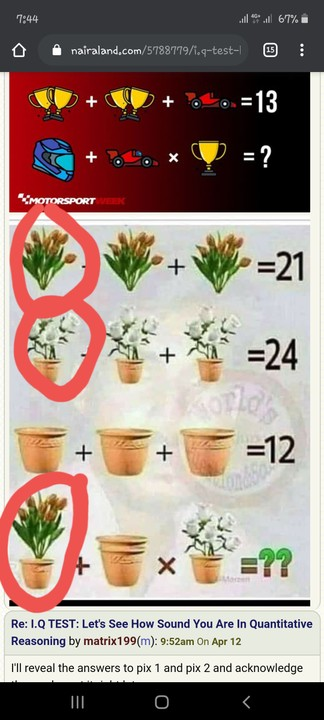Re: I.Q TEST: Let's See How Sound You Are In Quantitative Reasoning by CapedBaldy: 9:08am On Apr 13, 2020 20 & 39 Re: I.Q TEST: Let's See How Sound You Are In Quantitative Reasoning by Hallenjay: 9:15am On Apr 13, 2020 matrix199:I'll reveal the answers to pix 1 and pix 2 and acknowledge those who got it right later on. cc: RoyalRoyModified: I'm a very discreet person. The term 'acknowledge' means more than you might think. You never can tell! Puzzle 1For picture 1; let x represents the helmet. It implies that x + x + x = 30 3x = 30 x = 30/3 = 10 so x is 10For picture 2, let y represents the car. It implies that y + y+ x = 20 since x is 10 y + y + 10 = 20 2y + 10 = 20 2y = 20 - 10 2y = 10 y = 10/2 = 5 so y is 5For picture 3, let z represents the pair of trophies( 2 trophies). It implies that z + z + y = 13 since y is 5 z + z + 5 = 13 2z + 5 = 13 2z = 13 - 5 2z = 8 z = 8/2 = 4 so z is 4 But remember that z is a pair of trophies It implies that 1 trophy is 4/2 = 2For Picture 4, x(10) + y(5) x 1/2 of z(2) _____ 10 + 5 x 2Apply BODMAS _______________ 10 + (5 x 2) 10 + 10 = 20. Puzzle 2Take a good look at each quantitative picture. The 1st picture clearly shows 7 petals in each flower pot(7 + 7 + 7 = 21), discreetly indicating that each petal is 1. The 2nd picture also shows 4 roses in each flower pot. it implies that ( 4x2 + 4x2 + 4x2 =8 + 8 + 8= 24 or 12x2 = 24). The 3rd picture indicates that each empty pot is 4, that is ( 4 + 4 + 4 = 12). Now, the 4th picture shows a flower pot of 6 petals( each petal is 1, totalling 6 in combined value) + an empty flower pot having a value of 4 x a flower pot of 5 roses having a combined value of 10(since each rose is 2 in value) That is, 6 + 4 x 10 Don't forget to apply "BODMAS" 6 + (4 x 10) 6 + 40 = 46. Thus, for puzzle 1, the answer is 20! for puzzle 2, the answer is 46!Now take the correct explananation and answer. The first picture, first roll goes :helmet(h) +h+h=30>>3h/3=30/3. ....h=10 one helmet is equal to 10Second roll car(c) +c+h(10)=20>> 2c +10=202c/2=10/2 >> c=5I. E one car is equal to 5Third roll,different type of throphy +car =13Car is 5 which means d throphy altogether are 8.Now check the throphies, The one with dot has 3 base while the other with no dot has only one base. This simply means A trophy with 3 base rep 3 while the other is one. So in the 3rd roll, we have one 3 base throphy with one base +another 3 base with one base + the car. >> the 3base � and one base �(4)+3base � and one base �(4)+car(5)=13Now to the puzzle it sefOne helmet(10)+a helmet inside d car(15)×a throphy with one base. This simply means 10+15×1Applying BODMAS=15+10>>=25.--------------For the second puzzleThe petals are 7 in number with no pot7+7+7=21The second roll, four flower inside a pot. Automatically d pot rep 4 while d flowers are 4 each in a pot tooI.e 8+8+8=24THIRD Roll, empty potsPot+pot+pot =123pot/3=12/3>> a pot =4Forth roll, a flower pot with 6petals(10) + two pots (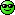(dats d view u Av when u placed one pot inside d other) ×five flower inside a pot(9)This simply means10+8×9Bodmas8×9=7272+10=82Therefore the first picture is 25While the second is 82.I await ur counter argument. *Before you ask me why I didn't multiply two pots or any object together instead of d addition.**Take a clue from the answered rolls,e.g four flower in a pot.**They didn't multiply d four flower by two and assume pot is two instead they add the flower value to the pot value which is 4+4 making 8 for each flowervace in 3 places which gives them 24.*cc: tocynone Re: I.Q TEST: Let's See How Sound You Are In Quantitative Reasoning by Richy4(m): 10:50am On Apr 13, 2020 matrix199:It's a play of trick. That the pot has two ring rigs doesn't allude it's doubled. The point is about the value of its emptiness. The alteration in the 4th picture of the empty pot was to create skepticism I like the way you were trying desperately to convince yourself that those empty flowers pots were not doubled. The pattern of the pot has been given. There's no need to trick anyone in changing the pattern or making a ring rigs as u call it... The way you figured out that the yellow trophies were no longer two but one, use that same logic on the flower pot. It's that simple. There's no benefit arguing this anyways. Have a good day man.. 1 Like Re: I.Q TEST: Let's See How Sound You Are In Quantitative Reasoning by ibkayee(f): 12:23pm On Apr 13, 2020 matrix199:It's a play of trick. That the pot has two ring rigs doesn't allude it's doubled. The point is about the value of its emptiness. The alteration in the 4th picture of the empty pot was to create skepticism You are having a laugh mate. Anywho, this is how I got my answerFirst:30/3 = 10(20-10)/2=5(13-5)/2=410 + (5+10) * (4/2)10 + ((15) * (2))10 + (30)= 40Things to note:4th row, 1st picture - No difference, helmet is 104th row, 2nd picture - There is a helmet on the racecar, so it becomes 10 (helmet) + 5 (racecar)4th row, 3rd picture - Only 1 cup, so divide 4 by 2Second:21/3 = 724/3 = 812/3 = 4(6+4) + (4x2) * (5+4)(10) + ((* (9))10 + (72)= 82Things to note:4th row, 1st picture - 6 flowers instead of 7 + a pot4th row, 2nd picture - 2 pots instead of 14th row, 3rd picture - 5 flowers instead of 4 Re: I.Q TEST: Let's See How Sound You Are In Quantitative Reasoning by matrix199(m): 3:07pm On Apr 13, 2020 Like I said earlier, it won't cost me anything to admit I was wrong. Controversy one....... the implication of the helmet in the car. I overlooked the mathematical operation ought to be involved(supposedly addition) because I presumed it was a decoyControversy two........ the implication of the two ring rigs. I overlooked the mathematical operation ought to be involved as well(supposedly addition) because I presumed it was also a decoy.So, let's give it another shot, but this time, taking the helmeted car and the two ring rigs into account.Puzzle 1.........Let x represent the helmet.x + x + x = 303x = 30x = 30/3 = 10so x(helmet) = 10Let y represent the cary + y + x = 202y + 10 = 202y = 20 - 10 = 10y = 10/2 = 5so y(car) = 5Let z represent the trophy(1 trophy)2z + 2z + y = 134z + 5 = 134z = 13 - 5 = 8z = 8/4 = 2so z(1 trophy) = 2Therefore. helmet(x) + a car and/with a helmet inside(x+y) x trophy(z)x + (y+x) x z = ?10 + (5+10) x 210 + [15 x 2]10 + 30 = 40.Puzzle 2......Take a good look at each quantitative picture. The 1st picture clearly shows 7 petals in each flower pot(7 + 7 + 7 = 21), discreetly indicating that each petal is 1. The 2nd picture also shows 4 roses in each flower pot. It implies that ( 4x2) + (4x2) + (4x2) =8 + 8 + 8= 24 or 12x2 = 24). It implies that 1 rose is 2 in value. The 3rd picture indicates each empty pot is 4, that is ( 4 + 4 + 4 = 12). Now, the 4th picture shows a flower pot of 6 petals( each petal is 1, totalling 6 in combined value) + (a doubled empty flower pot having a value of 4+4= 8 ) x (a flower pot of 5 roses having a combined value of 10(since each rose is 2 in value)) That is, 6 + 8 x 10 6 + (8x10) 6 + 80 = 86. Puzzle 1 is 40Puzzle 2 is 86 Re: I.Q TEST: Let's See How Sound You Are In Quantitative Reasoning by matrix199(m): 3:31pm On Apr 13, 2020 I appreciate everyone who was part of the trivia. For those who were a bit disrespectful, I understand you've not come of age. So I'll overlook the disrespect.Happy Easter all. Re: I.Q TEST: Let's See How Sound You Are In Quantitative Reasoning by Ardar(f): 3:48pm On Apr 13, 2020 First puzzle ~ 40Second puzzle ~ 86Wheew, the more you look the less you see(1) (2) (3) (4) (5) (Reply)

(Go Up)

 Sections: politics (1) business autos (1) jobs (1) career education (1) romance computers phones travel sports fashion health religion celebs tv-movies music-radio literature webmasters programming techmarket Nairaland - Copyright © 2005 - 2021 Oluwaseun Osewa. All rights reserved. See How To Advertise. 229Disclaimer: Every Nairaland member is solely responsible for anything that he/she posts or uploads on Nairaland.